## Hypothesis Testing

This Web page accompanies the article:

Anderson-Cook, C. M., and Dorai-Raj, S. (2003), "Making the Concepts of Power and Sample Size Relevant and Accessible to Students in Introductory Statistics Courses using Applets," Journal of Statistics Education [Online], 11(3). (jse.amstat.org/v11n3/anderson-cook.html)

In hypothesis testing we make a decision about the state of nature based on data. The following table represents the true state of nature (not known to the researcher) and the decision the researcher makes based on the data.

 State of Nature Null is true Alternative is true Decision fail toreject Null correctdecision Type IIerror reject Null Type Ierror correctdecision
From the table we see that there are two possibilities of error.
1. Type I : rejecting the null hypothesis when the null hypothesis is true.
2. Type II : failing to reject the null hypothesis when the null hypothesis is false.
where the probabilities of these errors are defined as follows: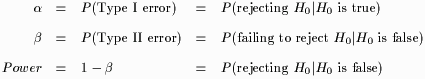Power will be the probability of being in the lower right cell of the table above. For a fixed Type I error rate () the goal of constructing and testing a hypothesis is to maximize Power. The purpose of these applets is to show how,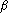and Power are related to each other. Power is affected by the following:

1.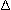, the difference in means between the null and alternative distributions,
2. n, the sample size, and
3., the Probability(Type I error).

The applet restricts the case to where the null and alternative distributions are both normal and only differ by a shift in the mean. Specifically,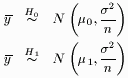where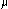0 is fixed at 10 and2 is fixed at 1.0. The hypothesis of interest will be the right-tailed hypothesis: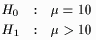Power is calculated using the following formula assuming2 is known:(1)
From (1), we see that Power is increased by
1. increasing n,
2. increasing, and
3. increasing.

It should be noted that in practice, increasingis not a practical way of increasing Power and is only mentioned here to demonstrate the relationship between the Type I and Type II error rates.

View Instructions for this applet
 -- Select an applet -- Change delta Change n Change alpha All of the above Open in new window   Open in current window